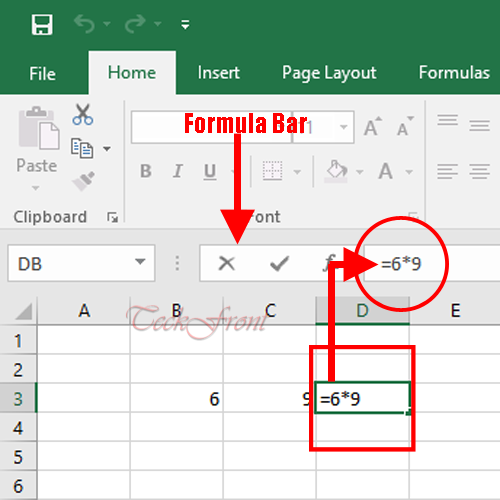The basic functions of easy and simple calculations in Microsoft Excel is performed with the help of the formulas. For the quick and routine calculations, many built-in tools and formulas are available in the Microsoft Excel. But if you are willing to calculate something which is beyond the working of the formulas, you may write your own formula.

Note: This tutorial is aimed to help the starters but not for the experts in Excel.

##### Basic Notation in the Working for Formulas – ‘=’

The basic notation in the formula creation and writing in Excel is sign of equal ‘=’. Whenever this notation is written and entered into any cell of Excel sheet, the other functions of Excel about the writing will be disabled immediately. Without entering the ‘=’ sign, you cannot write the formula in Excel cell.

1. Add 2 values in 2 cells, B2 and C2, of Excel worksheet.
2. In the 3rd cell – ‘D2’ – add ‘=’ sign.
3. After ‘=’ sign, write the 2 cell numbers, i.e. (in this situation) B2+C2.
4. See the output addition (SUM) is displayed in cell ‘D2’.
5. Alternatively, this calculation can be written as: ‘=2+4’
##### Minus, Multiplication and Divide Calculations

Just like above Addition function, simple calculation may be made for the following:

• Subtraction / Minus
• Multiplication
• Division calculation can be done on Excel sheet.

Note: Whenever you will enter formula in any cell of worksheet, it will be visible in the Formula Bar.

#####Return of Value

If you have entered some value in B3 cell and want to enter the same value in any other cell, just write ‘=B3’ and the same value will be produced in this cell though no calculation is performed in this function.

1. To reproduce any value from the range, select one value. I selected ’78’ from cell ‘B5’.
2. To reproduce the same in the ‘E7’, I entered the formula, ‘=B5’ and entered.
3. See the same 78 in ‘B5’ is reproduced in ‘E7’ though calculation is performed.
##### Adding All Values in Ranges

If you are willing to add all the values in one ranges, just apply the formula ‘=SUM(B1:B12) and all the values will be added.

1. See; in the range in cell ‘B’ is from ‘B1’ to ‘B7’.
2. Total of all the 7 values in the range are ‘256’ which is produced in cell ‘B8’.
3. Now, enter the formula ‘=SUM(B1:B7) and enter.
4. See; the output value in the cell ‘E6’ is same as was the total present in the cell ‘B8’.
##### Comparing 2 Values
1. If you want to compare 2 values, in any 2 cells, – say; values of B1 and C1 – apply this formula. ‘=B1=C1’
2. If the 2 values are equal, the result will be TRUE or Excel will return FALSE.
3. In this situation, 67 and 22 were not equal, the return was ‘FALSE’.